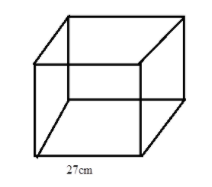Find the surface area of the wooden box which is in the shape of a cube, If the edge of the box is 27 cm.Verified
146.4k+ views
Hint: Use the formula of the surface area of a cube: surface area $= 6{a^2}$
Where a is the length of the side of the cube. Substitute the given length of the box in the formula to compute the surface area.

Complete step by step solution: We know that the formula for the surface area of a cube (Wooden box) of side length $a$ is given by
Surface area $= 6{a^2}$
Given that Length of box $= 27{\text{cm}}$
The cubical wooden box will look like the following figure:Therefore, the surface area of the wooden box
$= 6{\left( {27} \right)^2}$
$= 6 \times 729$
$= 4374{\text{c}}{{\text{m}}^2}$
Hence the answer is $4374{\text{c}}{{\text{m}}^2}$

Note: Each face of a cube is square in shape and a cube has 6 such faces. Therefore, the surface area of any cube-shaped object is the sum of the areas of each of its 6 faces.